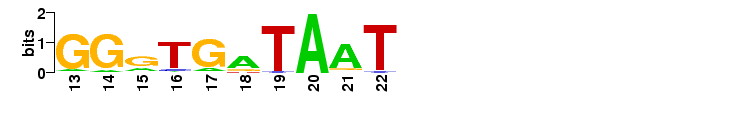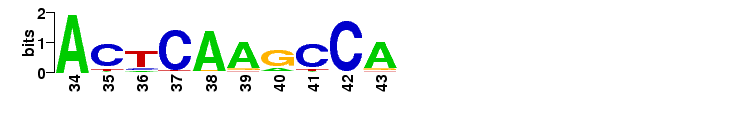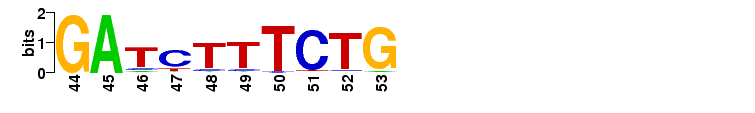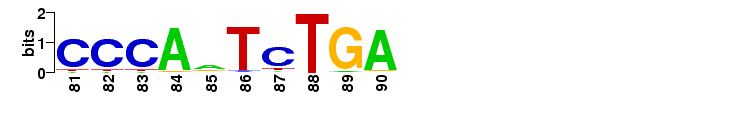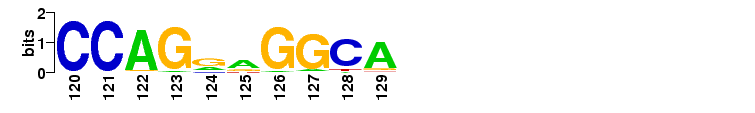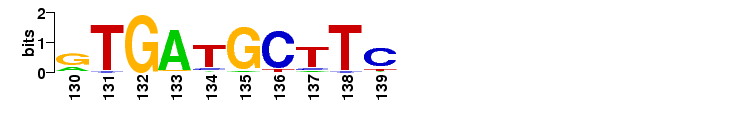# Example data set

This example illustrates the input and output of the maxAlike web server. We use a multiple sequence alignment based on one of the multiz 44-way alignments of homo sapiens (hg18) and other vertebrate species. From the 19 sequences in this particular alignment, we want to reconstruct the most likely sequence for the dog (denoted as canFam2 in the phylogenetic tree).

## Input

### Sequence Alignment

The alignment in Stockholm format looks like this (only first columns are shown)

```# STOCKHOLM 1.0
hg18                ATCATTATAACTGGCATTCTAATTGTAAAAAGAAACTTAAGGCAGATCTTTCTGGACAATAAC
micMur1             ACCATTATAGCTGGGTTTCTAATTGTAAAAACAAACTCAAGCCAGATTTTCCTGGACAATAAC
otoGar1             AGACTTTTAGCATGGATTCTAATTGGGAAAAGACACTCAAACCAGATCTTCCTGGACAATAAC
tarSyr1             ATAATTATAGCTGCGATTATAATTATAAAAAGAAACTCAAGCCAGATCTCTCTGGACAATCAC
calJac1             ATCATTATAACTGGCAACATAATTGTAAAAAGAAACGGCATGCAGAGCCTTCTGGTCAATAAC
rheMac2             ATCATTATAACTGGCATTCTAATTGTCAAAAGAAACATAAGGCAGATCTTTCTGGACAATAAC
ponAbe2             ATCATTATAACTGACATTCTAATCGTAAAAAGAAACTTAAGGCAGATCTTTCTGGACAATAAC
gorGor1             ATCATTATAACTGGCATTCTAATTGTAAAAAGAAACTTAAGGCAGATCTTTCTGGACAATAAC
panTro2             ATCATTATAACTGGCATTCTAATTGTAAAAAGAAACTTAAGGCAGATCTTTCTGGACAATAAC
dipOrd1             ACCATTGTGGCAAGGAATATAACTGTAAAAAGAAACTCAAATCAGATTTTTCTGGAAAAAAAA ...
speTri1             ATTATTATAGTTAGGATTATACTTACAAAAAGAGATTCAGGCCAGAGTTTTTCAGGAAAAGAA
oryCun1             ATCGTGATCATTGGAATCCTAATTGTTAGAGAAGACACAACCCCGATCATTCTGGAAAAGAAC
ochPri2             ACCATGACAGACAGAATTAAAATGATTAGAAAAGATACAGTCCGGATCATTCTGGACAGTAAT
cavPor3             ACTATTGCATTTAGGAGTATAATTGCTCAGAGACACTCAAGTCAGATTTCTCCAGCAGATAAA
turTru1             ACCGTCATAGCTAGGGTGATAATTATAAAACGAAATTCAAGCCAGATATTTCTGGAAAATAAC
bosTau4             ACTGTTATAGCCAGGGTGATAGTTATAAAAAGGAACTCAAACCAGACCTTTCTGAGAAATAAC
equCab2             ACTATTATAGCTAGGGTGATAATTATAAAAATAAACTCAAGCCAGATCTTTCTGGAAAATAAC
loxAfr2             ACCATTATAGCTGGGTTGATAATTAATAAAAAAAACTCAAGCCAGATTTATCTGTGAAATAAC
proCap1             ACTACCATAGCTGAACTGATAATTAATAAACAAAAAACAAGGTAGACTCATTTGTGAAATCTC
//
```

### Phylogenetic tree

The phylogenetic tree of all 44 species in Newick format looks like this:

```(((((((((((((((
((hg18:0.006591,panTro2:0.006639):0.002184,gorGor1:0.009411):0.009942,
ponAbe2:0.018342):0.014256,rheMac2:0.036199):0.021496,
calJac1:0.066389):0.056911,tarSyr1:0.135169):0.011307,
(micMur1:0.091452,otoGar1:0.128984):0.035463):0.015304,
tupBel1:0.183583):0.004688,(((((mm9:0.083220,rn4:0.090564):0.196605,
dipOrd1:0.209532):0.022555,cavPor3:0.223415):0.009828,
speTri1:0.146894):0.025042,
(oryCun1:0.116009,ochPri2:0.198295):0.100037):0.015355):0.020666,
(((vicPac1:0.105252,(turTru1:0.064182,bosTau4:0.121911):0.025111):0.039691,
((equCab2:0.107726,(felCat3:0.097971,canFam2:0.100888):0.049486):0.006252,
(myoLuc1:0.141155,pteVam1:0.111787):0.033187):0.004179):0.011699,
(eriEur1:0.220580,sorAra1:0.266859):0.056117):0.021065):0.023276,
(((loxAfr2:0.083775,proCap1:0.152633):0.026190,echTel1:0.240221):0.049905,
(dasNov2:0.115179,choHof1:0.096272):0.052373):0.006713):0.232748,
monDom4:0.325899):0.072430,ornAna1:0.453916):0.109903,
((galGal3:0.166386,taeGut1:0.170717):0.199763,
anoCar1:0.509545):0.108130):0.166150,xenTro2:0.852482):0.300396,
(((tetNig1:0.224774,fr2:0.205294):0.191836,
(gasAcu1:0.313967,oryLat2:0.478451):0.058404):0.322824,
danRer5:0.731166):0.155214):0.511293,petMar1:0.511293);```

Note that the names in the tree must match the names in the alignment file.

### Target species

Since we want to reconstruct the most likely sequence of the dog, we use canFam2 as the target name.

## Output

### Nucleotide probabilities

The main output file contains one line for each alignment column (only first 20 lines are shown).

```0	pair=-1	max_mu=0	IC=2.32193	A=1	G=0	C=0	T=0	-=0
1	pair=-1	max_mu=2.11763	IC=1.16445	A=0.0674266	G=0.0437362	C=0.753695	T=0.135142	-=0
2	pair=-1	max_mu=3.56614	IC=0.678139	A=0.110643	G=0.0717678	C=0.508599	T=0.30899	-=0
3	pair=-1	max_mu=2.18847	IC=0.943766	A=0.644017	G=0.240088	C=0.051334	T=0.0645603	-=0
4	pair=-1	max_mu=0.457851	IC=1.91297	A=0.0171434	G=0.01112	C=0.0305824	T=0.941154	-=0
5	pair=-1	max_mu=1.49823	IC=1.37601	A=0.0529735	G=0.0343613	C=0.0931442	T=0.819521	-=0
6	pair=-1	max_mu=1.68144	IC=1.2241	A=0.770531	G=0.13305	C=0.0427074	T=0.0537113	-=0
7	pair=-1	max_mu=0.935887	IC=1.63752	A=0.0341254	G=0.0221354	C=0.0587983	T=0.884941	-=0
8	pair=-1	max_mu=1.27804	IC=1.37449	A=0.81497	G=0.108736	C=0.0337935	T=0.0425006	-=0
9	pair=-1	max_mu=1.04112	IC=1.58642	A=0.0936975	G=0.864318	C=0.0185963	T=0.0233878	-=0
10	pair=-1	max_mu=1.63799	IC=1.32655	A=0.0530898	G=0.0344366	C=0.803132	T=0.109341	-=0
11	pair=-1	max_mu=2.18761	IC=1.16107	A=0.0745705	G=0.04837	C=0.119688	T=0.757371	-=0
12	pair=-1	max_mu=2.34882	IC=0.824536	A=0.520819	G=0.362756	C=0.0515687	T=0.0648559	-=0
13	pair=-1	max_mu=0.933621	IC=1.63947	A=0.0847809	G=0.877595	C=0.0166649	T=0.0209587	-=0
14	pair=-1	max_mu=1.07897	IC=1.56838	A=0.096802	G=0.859678	C=0.0192765	T=0.0242432	-=0
15	pair=-1	max_mu=2.67404	IC=0.91067	A=0.268895	G=0.61654	C=0.050638	T=0.0639265	-=0
16	pair=-1	max_mu=1.59647	IC=1.35679	A=0.056158	G=0.0364268	C=0.0923249	T=0.81509	-=0
17	pair=-1	max_mu=1.27631	IC=1.47305	A=0.112811	G=0.834471	C=0.0229354	T=0.0297822	-=0
18	pair=-1	max_mu=2.86178	IC=0.919825	A=0.664303	G=0.185951	C=0.0671282	T=0.0826174	-=0
19	pair=-1	max_mu=0.47544	IC=1.90122	A=0.0177845	G=0.0115359	C=0.0316836	T=0.938996	-=0
```

### Sequence logo

The probabilities are shown in the sequence logo: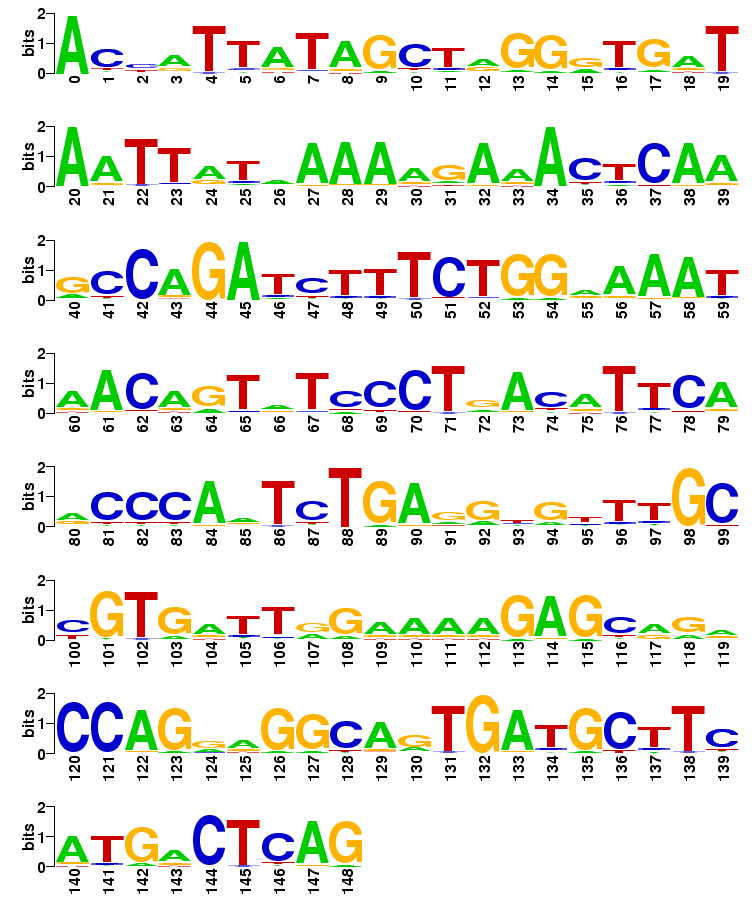### Consensus sequences

Predicted consensus sequence, with a probability cutoff 0.5 at each site:

>canFam2 predicted consensus sequence with probability cutoff 0.5
ACCATTATAGCTAGGGTGATAATTATAAAAAGAAACTCAAGCCAGATCTTTCTGGAAAATAACAGTATCCCTGACATTCA
ACCCAATCTGAGGTGTTTGCCGTGATTGGAAAAGAGCAGACCAGGAGGCAGTGATGCTTCATGACTCAG

Predicted consensus sequence, without cutoff:

>canFam2 predicted consensus sequence
ACCATTATAGCTAGGGTGATAATTATAAAAAGAAACTCAAGCCAGATCTTTCTGGAAAATAACAGTATCCCTGACATTCA
ACCCAATCTGAGGTGTTTGCCGTGATTGGAAAAGAGCAGACCAGGAGGCAGTGATGCTTCATGACTCAG

### Conserved Elements

Subsequences having a window length of 10nt with average information content above 1.5: